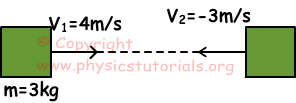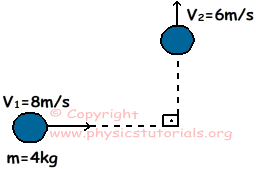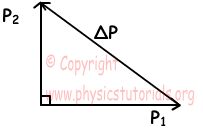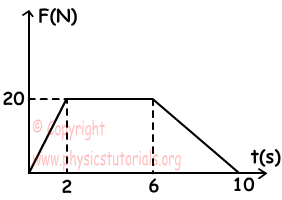Impulse Momentum Exam1 and Problem Solutions

Impulse Momentum Exam1 and Problem Solutions

1. An object travels with a velocity 4m/s to the east. Then, its direction of motion and magnitude of velocity are changed. Picture given below shows the directions and magnitudes of velocities. Find the impulse given to this object.I=F.Δt=Δp=m.ΔV

where ΔV=V2-V1=-3-4=-7m/s

I=m.ΔV=3.(-7)=-21kg.m/s

2. Ball having mass 4kg and velocity 8m/s travels to the east. Impulse given at point O, makes it change direction to north with velocity 6m/s. Find the given impulse and change in the momentum.Initial and final momentum vectors of ball are shown in the figure below.P1=m.V1=4kg.8m/s=32kg.m/s

P2=m.V2=4kg.6m/s=24kg.m/s

ΔP2=P22+P12=m2(v22+v12)

ΔP2=16.100 ΔP=40kg.m/s Impulse=change in momentum

I=ΔP=40kg.m/s

3. Find the impulse and force which make 12m/s change in the velocity of object having 16kg mass in 4 s.

F.Δt=ΔP=m.ΔV

F.4s=16kg.12m/s

F=48N

F.Δt=Impulse=192kg.m/s

4. Applied force vs. time graph of object is given below. Find the impulse of the object between 0-10s.Area under the force vs. time graph gives us impulse.

F.Δt=20.2/2+20.(6-2)+20.(10-6)/2

F.Δt=140kg.m/s

5. A ball having mass 500g hits wall with a10m/s velocity. Wall applies 4000 N force to the ball and it turns back with 8m/s velocity. Find the time of ball-wall contact.

F.Δt=ΔP=m.ΔV=m.(V2-V1)

-4000.Δt=0,5kg.(8-10)

Δt=0,00025s

Author: